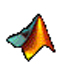The Utility Toolbox contains functions to manipulate data frames or do basic useful data manipulations in general.

### Data Frames

Data is stored in a data frames, the equivalent of a spreadsheet in Excel, SPSS, and corrsponding (and inspired by) Data Frames in R. Spread sheets are often saved in tab-delimited text files, with the first column referring to the names of variables. The util-toolbox contains functions to read these files. In Matlab, a data frame is represented in a structure containing fields corresponding to the variable names. Each contains the data column below. These data fields can contain numerical values, strings (stored as cell array), or even row-vectors of the same length (for example time series).

Data FileCommandData structure in workspace
 Age Initials Haircolor Weight 23 DF brown 120 17 AT blond 163 44 CF black 180 32 KT blond 187 ... ... ... ...

---->

D =
Age: [6x1 double]
Initials: {6x1 cell}
Haircolor: {6x1 cell}
Weight: [6x1 double]

Most of the subsequent tabulation and statistical graphing functions work with these data frames. The util toolbox contains useful functions for the manipulation of data frames (inserting rows, extracting rows, etc)

### Variable function parameters

Another concept borrowed from R is the felxible way of passing input parameters. Nearly all functions use a variable input structure. This means that you can pass the parameters to these functions in any number or sequence. Thus, you don't have to remember the exact sequence of the input arguments. You simply specify the name of the parameter before each parameter you want to pass. For example:
myfunction('option1',value1,'option2',value2,'flag1','option3',value3);
calls the function myfunction and sets the variables option1, option2, option3 to their respective values. It also sets the flag flag1 to TRUE. Use help myfunction to determine valid options and flags for each function.

## List of functions

 1. Data Structure functions dload Loads data file into data structure (field or variable names are given my first row) dsave Save a data structure as a data file with headers (ASCII) dprint Prints data structure on screen dshuffel Randomizes the rows of a data structure addstruct Adds a data structure to another - field by field getrow Returns rows x from a data structure setrow Sets rows x in a data structure insertrow Insert row(s) at position x into a data structure field_matrix2vector Changes a matrix into fields of column vectors, called var1,var2,... field_vector2matrix Changes vectors var1,var,... back into matrix var 2. Other Data manipulations findrow Finds a row of certain values in a Matrix findIdx Finds indices based on several criteria combined by and/or minmax Finds absolute minimum/maximum nancount Returns number of nans in a vector fisherz Fisher-z transformation of correlation fisherinv Inverse of fisher-z split_data Splits ordinal data into p categories 3. Input/output functions get_response Waits for a user-key press vararginoptions Deals with argument lists passed to functions fcneval Evaluates a function argument with eval or feval struct2list Makes a structure into a argument listJörn Diedrichsen'sHomepageMatlabToolboxes